# A menagerie of infinite matroids

So far in this series we have focused on the search for a good way to axiomatise infinite matroids. Now that we have the axioms, our next aim is to get a better sense of what sort of ground they stake out by taking a whirlwind tour of the known examples of infinite matroids.

Let’s start off with some very familiar examples: The set of subsets of a given set which have size at most $n$,  the set of (edge sets of) forests in a given graph and the set of linearly independent subsets of a given family of vectors in a vector space each give the set of independent sets of a matroid. This is true even if we allow the set, graph or family of vectors in question to be infinite. Of course, all of these examples are finitary (that is, a set is independent as soon as all of its finite subsets are). We saw earlier that it would be a bad idea to only consider finitary matroids, because we would lose the power of duality. But we certainly want our notion of infinite matroid to include all of these examples. Since we now have a concept of infinite matroid which is closed under duality, we get all their duals for free, too.

What about matroids which are neither finitary nor cofinitary? At first it looks like a simple way to construct such examples would be by building infinite uniform matroids. But the usual definition of uniformity, namely that the bases should be all sets of a given size, becomes silly when we replace size’ by cardinality’, since infinite sets can have proper subsets of the same cardinality. It doesn’t help to define things in terms of independent sets. For example, we might hope to have a matroid whose independent sets are just the countable subsets of some uncountable set $E$. But since there are no maximal countable sets, this object wouldn’t even have any bases.

The right notion of uniformity for infinite matroids is as follows: if $B$ is a base and $x$ and $y$ are elements of the ground set $E$ with $x$ in $B$ but $y$ not in $B$ then $B – x + y$ is also a base. It isn’t hard to check that this is equivalent to uniformity when applied to finite matroids. Now we might try to build infinite uniform matroids by just recursively choosing the set of bases, paying attention to the fact that (because of the definition above) whenever we choose a set $B$ we also have to choose all sets $B’$ for which $B \setminus B’$ and $B’ \setminus B$ are finite and of equal size. This simpleminded strategy works, given some weak assumptions [BG15]. Because this recursive construction gives us so much freedom, infinite uniform matroids are much more varied than finite ones.

The flexibility of this construction lets us resolve a very basic question: must it be true that any two bases of an infinite matroid have the same cardinality? Higgs showed that this is true if we assume the continuum hypothesis [H69a]. But using the freedom mentioned above we can also show that it is consistent that the following naive plan for building a matroid with bases of different sizes actually works: run the above recursive construction to build a uniform matroid on an uncountable set, and begin by nominating some countable subset and some uncountable subset as bases [BG15]. So the answer to the question above is actually independent of the usual axioms of set theory.

The other examples we’ll look at today will all be associated to graphs. The finitary matroid mentioned above, whose circuits are (edge sets of) cycles in a given graph $G$, is called the finite-cycle matroid of $G$. There is another finitary matroid whose circuits are the finite bonds of $G$. But these two are no longer dual to each other. The circuits of the dual of the finite-bond matroid correspond to topological circles in a topological space, called the end-compactification of $G$. This space is obtained from $G$ by adding some points at infinity, called ends. These `topological cycles’ were actually first invented for a very different reason and helped to motivate the current axiomatisations of infinite matroids. This was the starting point of our story.

So although there is one canonical matroid associated to a finite graph, for an infinite graph we already have two. In fact there are many more. For example, Higgs showed that there very often is a matroid whose circuits are the (edge sets of) finite cycles or double rays in the graph $G$. This doesn’t always work. For example, in the Bean Graph, shown below, the set of bold edges and the set of dashed edges are both bases but there is no way to replace the edge $e$ with a bold edge in the dashed basis and still have a base. So the base axioms don’t hold.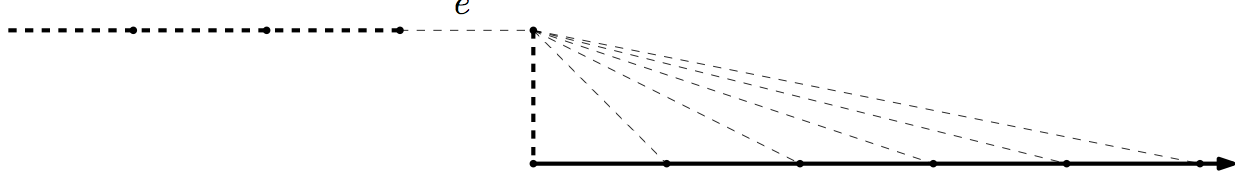This is essentially all that can go wrong: Higgs showed [H69b] that if $G$ includes no subdivision of the Bean Graph then there is a matroid, called the algebraic-cycle matroid of $G$, whose circuits are given as above by the finite cycles and double rays.

Another fertile way to build more matroids from graphs is to ignore some of the ends. More precisely, we consider topological circles in a space obtained from the end-compactification of $G$ by deleting some of the ends. For example, the double ladder has two ends and we might wish to keep only one of them, giving rise to the following space: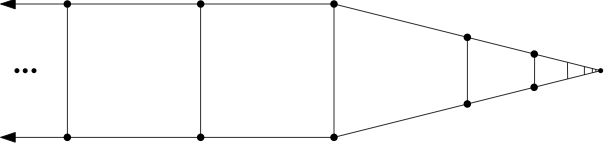Once more it is true that the (edge sets of) topological circles give the circuits of a matroid, as long as the set of ends we remove is Borel [BC15].

Is there any unifying theme to this profusion of examples, except that they can all be built somehow from graphs? Yes! Each of these matroids can represented by a topological space. The finite-cycle matroid of $G$ is represented by the geometric realisation of $G$ and the topological-cycle matroid by the end-compactification. The rich collection of matroids we just discussed are represented by subspaces of the end-compactification. The algebraic-cycle matroid is represented by the space obtained from the end-compactification by identifying all the ends.

More generally, there is a precisely specified class of graph-like topological spaces and a  notion of representation of a matroid by such a space, such that a matroid is representable in this way if and only if, firstly, every intersection of a circuit with a cocircuit is finite (this corresponds to the fact that topological circles are compact), and secondly, it satisfies the usual excluded-minor characterisation of graphic matroids.

Thus the wide range of ways of building infinite matroids from graphs corresponds to the variety of ways of building such graph-like spaces out of graphs. We have seen that this construction doesn’t always give a matroid, as with the Bean Graph above, but it does so often enough to give a rich variety of matroids. For example, we always get a matroid when the space is compact. Hence the (edge sets of) topological circles in the following picture give the circuits of a matroid, the Sierpinski Matroid: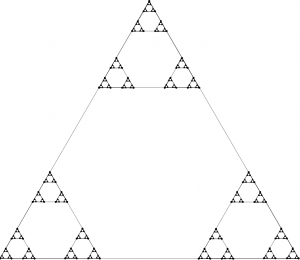Before we conclude, lets just glance at a couple of the other kinds of infinite matroid which have been discovered. There are frame matroids: Matthews and Oxley showed that in any graph $G$ the edge sets of subdivisions of the following graphs gives the set of circuits of a matroid [MO77], and a more general construction works in in infinite biased graphs.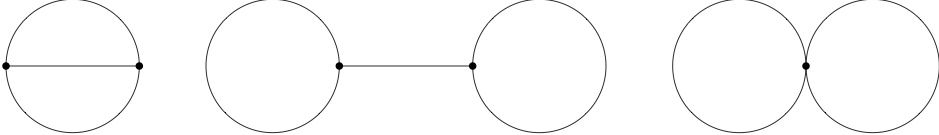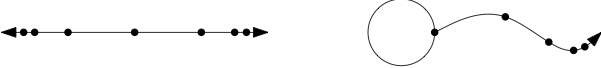There are infinite gammoids, defined either in terms of bipartite graphs or of directed graphs. The question of when these constructions give rise to matroids is a delicate one and has been investigated by Afzali, Law and Müller [ALM15].

Finally, we can define the truncation of a non-free matroid $M$ to have as independent sets the proper subsets of independent sets of $M$, and all truncations and co-truncations of infinite matroids are again infinite matroids. This gives lots of new examples, such as the matroids whose circuits are edge sets of topological copies of the following spaces in a graph-like space: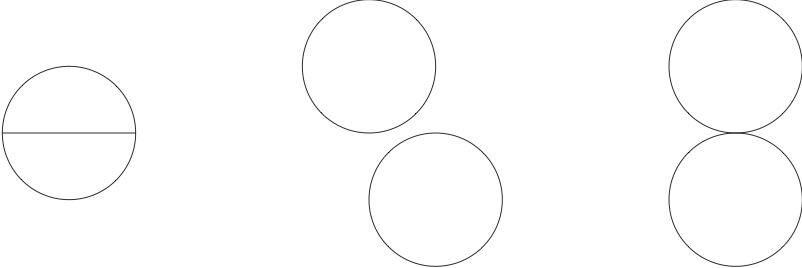This is not so much a class of examples as a way to build new infinite matroids from old. We’ll consider some other fertile ways to combine infinite matroids next time.

[ALM15] H. Afzali, Hiu-Fai Law and M. Müller, Infinite gammoids. Electronic J. Comb. 22 (2015), #P1.53
[BC15] N. Bowler and J. Carmesin, Infinite Matroids and Determinacy of Games, submitted to LMS, available here.
[BG15] N. Bowler and S. Geschke,  Self-Dual Uniform Matroids on Infinite Sets, to appear in the Bulletin of the American Mathematical Society, available here.
[H69a] D. Higgs, Equicardinality of bases in B-matroids, Canadian Math. Bul. 12 (1969), 861–862.
[H69b] D. Higgs. Infinite graphs and matroids. Recent Progress in Combinatorics, Proceedings Third Waterloo Conference on Combinatorics, Academic Press (1969),  245-53.
[MO77] L. Matthews, J. Oxley, Infinite graphs and bicircular matroids. Discrete Mathematics, Volume 19, Issue 1 (1977), 61-65.

This entry was posted in Matroids by Nathan Bowler. Bookmark the permalink.I'm working at the university of Hamburg on infinite matroid theory.

## 2 thoughts on “A menagerie of infinite matroids”

1.Naomi on said:

Hi, I wrote my master thesis about infinite matroids and how their poset of flats is a special infinite “geometric” lattice.
Formally everything seemed pretty clear to me, but when it comes to examples, I struggle with the infinity. What is the easiest infinite graphic lattice you can think of?

2.Nathan Bowler on said:

I’m afraid I need a little more context to answer this properly, since on the usual definition geometric lattices are necessarily finite and I have seen different approaches to defining an infinite version of this concept. Which were you working with in your masters thesis?

This site uses Akismet to reduce spam. Learn how your comment data is processed.